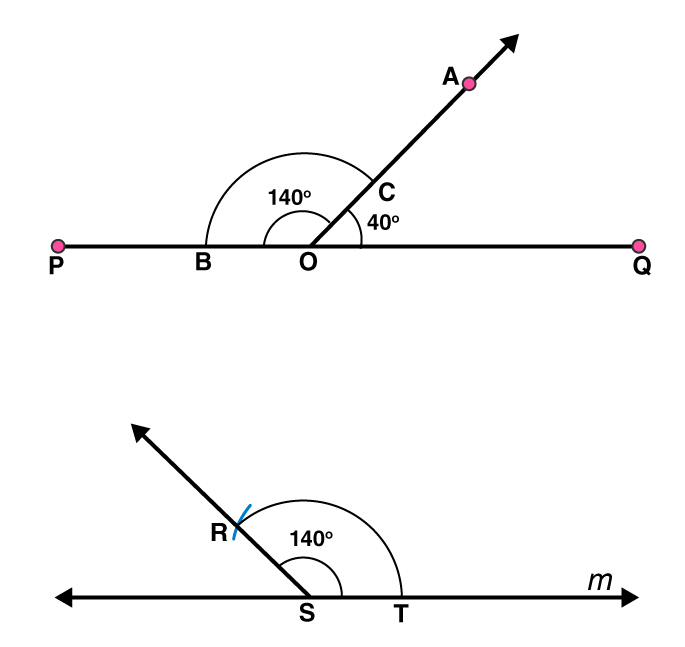## Access NCERT Solutions for Class 6 Chapter 14: Practical Geometry Exercise 14.6

### Access NCERT Solutions for Class 6 Chapter 14: Practical Geometry Exercise 14.6

1. Draw ∠POQ of measure 75° and find its line of symmetry.

Solutions:

Following steps are followed to construct an angle of 750 and its line of symmetry

(i) Draw a line l and mark two points O and Q on it. Draw an arc of convenient radius, while taking centre as O. Let this intersect line l at R

(ii) Taking R as centre and with same radius as before, draw an arc such that it is intersecting the previously drawn arc at S

(iii) By taking same radius as before and S as centre, draw an arc intersecting the arc at point T as shown in figure

(iv) Take S and T as centre, draw an arc of same radius such that they intersect each other at U

(v) Join OU. Let it intersect the arc at V. Now, take S and V as centres draw arcs with radius more than 1 / 2 SV. Let these intersect each other at P. Join OP. Now OP is the ray making 750 with the line l.

(vi) Let this ray intersect our major arc at point W. By taking R and W as centres, draw arcs with radius more than 1 / 2 RW in the interior angle of 750. Let these intersect each other at point X. Join OX

OX is the line of symmetry for the ∠POQ = 7502. Draw an angle of measure 147° and construct its bisector.

Solutions:

Following steps are followed to construct an angle of measure 1470 and its bisector

(i) Draw a line l and mark point O on it. Place the centre of protractor at point O and the zero edge along line l

(ii) Mark a point A at an angle of measure 1470. Join OA. Now OA is the required ray making 1470 with line l

(iii) By taking point O as centre, draw an arc of convenient radius. Let this intersect both rays of angle 1470 at points A and B.

(iv) By taking A and B as centres draw arcs of radius more than 1 / 2 AB in the interior angle of 1470. Let these intersect each other at point C. Join OC.

OC is the required bisector of 1470 angle3. Draw a right angle and construct its bisector.

Solutions:

Following steps are followed to construct a right angle and its bisector.

(i) Draw a line l and mark a point P on it. Draw an arc of convenient radius by taking point P as centre. Let this intersect line l at R

(ii) Draw an arc by taking R as centre and with the same radius as before such that it is intersecting the previously drawn arc at S

(iii) Take S as centre and with the same radius as before, draw an arc intersecting the arc at T as shown in figure

(iv) By taking S and T as centres draw arcs of same radius such that they are intersecting each other at U.

(v) Join PU. PU is the required ray making a right angle with the line l. Let this intersect major arc at point V.

(vi) Now take R and V as centres, draw arcs with radius more than 1 / 2 RV to intersect each other at point W. Join PW.

PW is the required bisector of this right angle.4. Draw an angle of measure 153° and divide it into four equal parts.

Solutions:

Following steps are followed to construct an angle of measure 1530 and its bisector

(i) Draw a line l and mark a point O on it. Place the centre of protractor at point O and the zero edge along line l

(ii) Mark a point A at the measure of angle 1530. Join OA. Now OA is the required ray making 1530 with line l

(iii) Draw an arc of convenient radius by taking point O as centre. Let this intersect both rays of angle 1530 at points A and B.

(iv) Take A and B as centres and draw arcs of radius more than 1 / 2 AB in the interior of angle of 1530. Let these intersect each other at C. Join OC

(v) Let OC intersect major arc at point D. Draw arcs of radius more than 1 / 2 AD with A and D as centres and also D and B as centres. Let these are intersecting each other at points E and F, respectively. Now join OE and OF

OF, OC, OE are the rays dividing 1530 angle into four equal parts.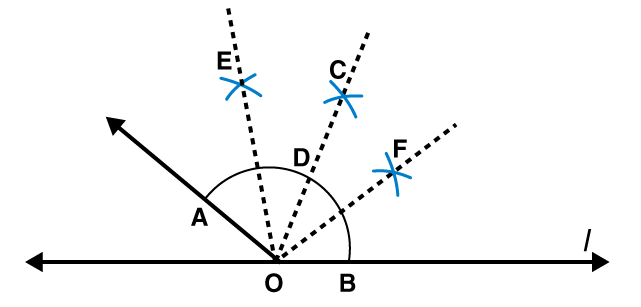5. Construct with ruler and compasses, angles of following measures:

(a) 60°

(b) 30°

(c) 90°

(d) 120°

(e) 45°

(f) 135°

Solutions:

(a) 600

Following steps are followed to construct an angle of 600

(i) Draw a line l and mark a point P on it. Take P as centre and with convenient radius, draw an arc of a circle such that it intersects the line l at Q.

(ii) Take Q as centre and with the same radius as before, draw an arc intersecting the previously drawn arc at point R.

(iii) Join PR. PR is the required ray making 600 with the line l.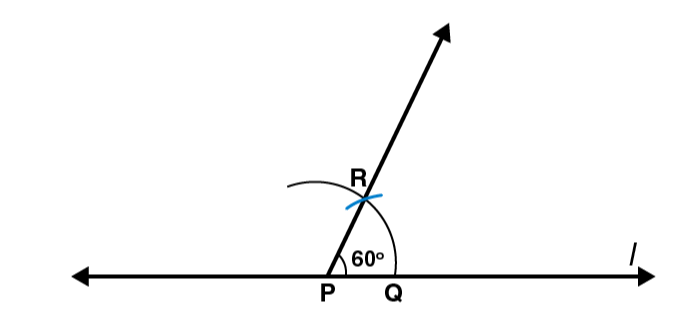(b) 300

Following steps are followed to construct an angle of 300

(i) Draw a line l and mark a point P on it. By taking P as centre and with convenient radius, draw an arc of a circle such that it is intersecting the line l at Q.

(ii) Take Q as centre and with the same radius as before, draw an arc intersecting the previously drawn arc at point R.

(iii) By taking Q and R as centres and with radius more than 1 / 2 RQ draw arcs such that they are intersecting each other at S. Join PS which is the required ray making 300 with the line l.(c) 900

Following steps are followed to construct an angle of measure 900

(i) Draw a line l and mark a point P on it. Take P as centre and with convenient radius, draw an arc of a circle such that it is intersecting the line l at Q.

(ii) Take Q as centre and with the same radius as before, draw an arc intersecting the previously drawn arc at R

(iii) By taking R as centre and with the same radius as before, draw an arc intersecting the arc at S as shown in figure

(iv) Now take R and S as centre, draw arc of same radius to intersect each other at T.

(v) Join PT, which is the required ray making 900 with the line l.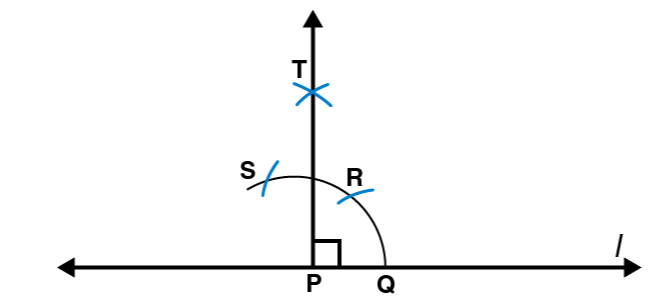(d) 1200

Following steps are followed to construct an angle of measure 1200

(i) Draw a line l and mark a point P on it. Taking P as centre and with convenient radius, draw an arc of circle such that it is intersecting the line l at Q.

(ii) By taking Q as centre and with the same radius as before, draw an arc intersecting the previously drawn arc at R.

(iii) Take R as centre and with the same radius as before, draw an arc such that it is intersecting the arc at S as shown in figure.

(iv) Join PS, which is the required ray making 1200 with the line l(e) 450

Following steps are followed to construct an angle of measure 450

(i) Draw a line l and mark a point P on it. Take P as centre and with convenient radius, draw an arc of a circle such that it is intersecting the line l at Q.

(ii) Take Q as centre and with the same radius as before, draw an arc intersecting the previously drawn arc at R

(iii) By taking R as centre and with the same radius as before, draw an arc such that it is intersecting the arc at S as shown in figure.

(iv) Take R and S as centres and draw arcs of same radius such that they are intersecting each other at T

(v) Join PT. Let this intersect the major arc at point U.

(vi) Now take Q and U as centres and draw arcs with radius more than 1 / 2 QU to intersect each other at point V. Join PV.

PV is the required ray making 450 with the line l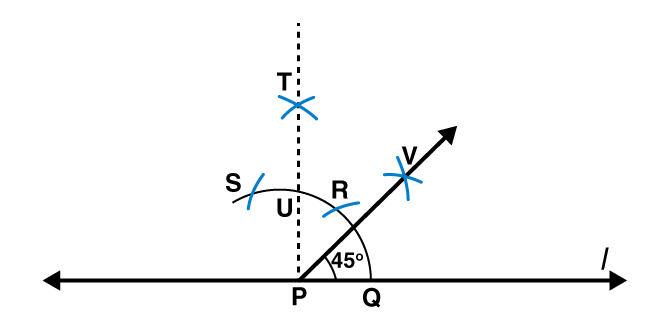(f) 1350

Following steps are followed to construct an angle of measure 1350

(i) Draw a line l and mark a point P on it. Taking P as centre and with convenient radius, draw a semicircle which intersects the line l at Q and R, respectively.

(ii) By taking R as centre and with the same radius as before, draw an arc intersecting the previously drawn arc at S

(iii) Taking S as centre and with the same radius as before, draw an arc such that it is intersecting the arc at T as shown in figure

(iv) Take S and T as centres, draw arcs of same radius to intersect each other at U.

(v) Join PU. Let this intersect the arc at V. Now take Q and V as centres and with radius more than 1 / 2 QV, draw arcs to intersect each other at W.

(vi) Join PW which is the required ray making 1350 with the line l6. Draw an angle of measure 45° and bisect it.

Solutions:

Following steps are followed to construct an angle of measure 450 and its bisector.

(i) Using the protractor ∠POQ of 450 measure may be formed on a line l

(ii) Draw an arc of convenient radius with centre as O. Let this intersect both rays of angle 450 at points A and B

(iii) Take A and B as centres, draw arcs of radius more than 1 / 2 AB in the interior of angle of 450. Let these intersect each other at C. Join OC

OC is the required bisector of 450 angle7. Draw an angle of measure 135° and bisect it.

Solutions:

Following steps are followed to construct an angle of measure 1350 and its bisector.

(i) By using a protractor ∠POQ of 1350 measure may be formed on a line l

(ii) Draw an arc of convenient radius by taking O as centre. Let this intersect both rays of angle 1350 at points A and B, respectively.

(iii) Take A and B as centres, draw arcs of radius more than 1 / 2 AB in the interior of angle of 1350. Let these intersect each other at C. Join OC.

OC is the required bisector of 1350 angle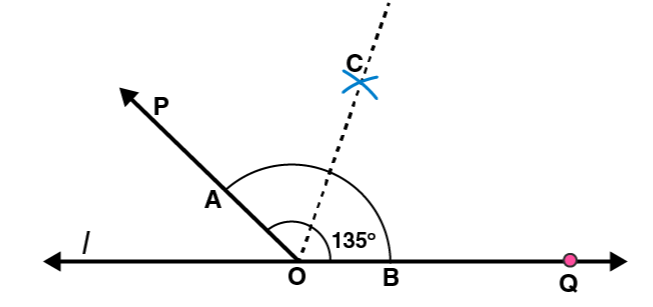8. Draw an angle of 700. Make a copy of it using only a straight edge and compasses.

Solutions:

Following steps are followed to construct an angle of measure 700 and its copy.

(i) Draw a line l and mark a point O on it. Now place the centre of protractor at point O and the zero edge along line l.

(ii) Mark a point A at an angle of measure 700. Join OA. Now OA is the ray making 700 with line l. With point O as centre, draw an arc of convenient radius in the interior of 700 angle. Let this intersect both rays of angle 700 at points B and C, respectively

(iii) Draw a line m and mark a point P on it. Again draw an arc with same radius as before and P as centre. Let it cut the line m at point D

(iv) Adjust the compasses up to the length of BC. With this radius draw an arc taking D as centre which intersects the previously drawn arc at point E.

(v) Join PE. Here PE is the required ray which makes same angle of measure 700 with the line m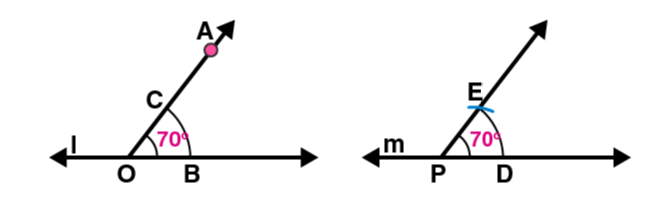9. Draw an angle of 400. Copy its supplementary angle.

Solutions:

Following steps are followed to construct an angle of measure 450 and a copy of its supplementary angle

(i) Draw a line segment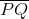and mark a point O on it. Place the centre of protractor at point O and the zero edge along line segment.

(ii) Mark a point A at an angle of measure 400. Join OA. Here OA is the required ray making 400 with. ∠POA is the supplementary angle of 400

(iii) With point O as centre, draw an arc of convenient radius in the interior of ∠POA. Let this intersect both rays of ∠POA at points B and C, respectively.

(iv) Draw a line m and mark a point S on it. Again draw an arc by taking S as centre with the same radius as used before. Let it cut the line m at point T.

(v) Now adjust the compasses up to the length of BC. Taking T as centre draw an arc with this radius which will intersect the previously drawn arc at point R.

(vi) Join RS. Here RS is the required ray which makes same angle with the line m, as the supplementary of 400 [i.e 1400]